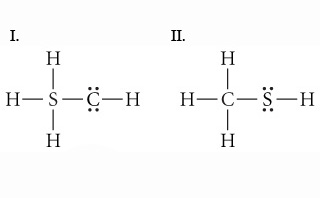Problem: Use formal charges to identify the better Lewis structure.

FREE Expert Solution
91% (133 ratings)
FREE Expert Solution

We have to determine which of the given Lewis structures is a better structure.

A better Lewis structure is the one which has the smallest possible formal charges on all the atoms in the molecule.

Formal charge is the charge assigned to an atom in a Lewis structure assuming that the electrons in the chemical bonds are shared equally between atoms.

Formal charge is calculated by this formula:

91% (133 ratings)
Problem DetailsUse formal charges to identify the better Lewis structure.

What scientific concept do you need to know in order to solve this problem?

Our tutors have indicated that to solve this problem you will need to apply the Formal Charge concept. You can view video lessons to learn Formal Charge Or if you need more Formal Charge practice, you can also practice Formal Charge practice problems .

What is the difficulty of this problem?

Our tutors rated the difficulty of Use formal charges to identify the better Lewis structure.... as low difficulty.

How long does this problem take to solve?

Our expert Chemistry tutor, Dasha took 3 minutes to solve this problem. You can follow their steps in the video explanation above.

What professor is this problem relevant for?

Based on our data, we think this problem is relevant for Professor Enderle's class at UCD.

What textbook is this problem found in?

Our data indicates that this problem or a close variation was asked in . You can also practice practice problems .